## GATE 2018

 Question 1

“From where are they bringing their books? __________ bringing __________ books from __________.”

The words that best fill the blanks in the above sentence are

 A Their, they’re, there B They’re, their, there C There, their, they’re D They’re, there, there
Aptitude       Verbal
Question 1 Explanation:Question 2

"A _______ investigation can sometimes yield new facts, but typically organized ones are more successful."

The word that best fills the blank in the above sentence is

 A meandering B timely C consistent D systematic
Aptitude       Verbal
Question 2 Explanation:
Meandering means "Proceeding in a convoluted way (or) undirected fashion".
 Question 3

The area of a square is d. What is the area of the circle which has the diagonal of the square as its diameter?

 A πd B πd2 C (1/2)πd2 D (1/2)πd
Aptitude       Numerical
Question 3 Explanation:
In general,
Area of a square A = a2 (where a is side)
In the question,
Area of a square = d
The side of a square = √d
Diagonal of a square = Diameter of circle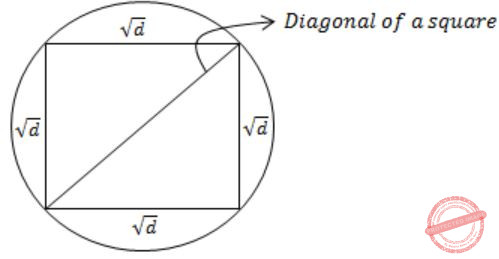From Pythagoras theorem,Question 4

What is the missing number in the following sequence?

2, 12, 60, 240, 720, 1440, _______, 0
 A 2880 B 1440 C 720 D 0
Aptitude       Numerical
Question 4 Explanation:2 × 6 = 12
12 × 5 = 60
60 × 4 = 240
240 × 3 = 720
720 × 2 = 14401440 × 0 = 0
 Question 5

What would be the smallest natural number which when divided either by 20 or by 42 or by 76 leaves a remainder of '7' in each case?

 A 3047 B 6047 C 7987 D 63847
Aptitude       Numerical
Question 5 Explanation:
Method-I:
Take the LCM of 20, 42, 76 i.e., 7980.
⟹ Remainder = 7
⟹ Smallest number divisible by 20 (or) 42 (or) 72 which leaves remainder 7 is = 7980 + 7 = 7987
Method-II:
Option I:
3047 – 7 = 3040
3040 not divisible by 42
Option II:
6040 not divisible by 42
Option III:
7980 divisible by 20, 42, 76
Option IV:
63840 divisible by 20, 42, 76
→ Smallest (7980, 63840) = 7980
→ 7980 + remainder 7 = 7987
 Question 6

If pqr ≠ 0 and p -x = 1/q, q -y = 1/r, r -z = 1/p, what is the value of the product xyz?

 A -1 B 1/pqr C 1 D pqr
Aptitude       Numerical
Question 6 Explanation:
pqr≠0
→ p-x = 1/q ⟹ 1/px = 1/q
⟹ q = px
⟹ log q = log px
⟹ x log p = log q
⟹ x = log q/ log p
→ q-y = 1/r ⟹ 1/qy = 1/r
⟹ qy = r
⟹ y log q = log r
⟹ y = log r/ log q
→ r-z = 1/p ⟹ 1/rz = 1/p
⟹ p = rz
⟹ log rz = log p
⟹ z log r = log p
⟹ z = log p/ log r
XYZ = log q/ log p * log r/ log q * log p/ log r =1
 Question 7

In the figure below, ∠DEC + ∠BFC is equal to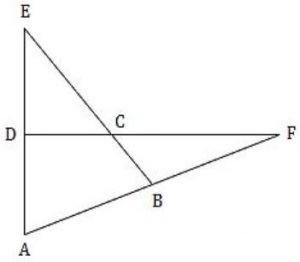A ∠BCD - ∠BAD B ∠BAD + ∠BCF C ∠BAD + ∠BCD D ∠CBA + ADC
Aptitude       Numerical
Question 7 Explanation:→ ∠1 = ∠5 + ∠4 ………(i)
According to triangular property:
Angle of exterior = sum of interior angles
→ ∠4 = ∠3 + ∠2 ……….(ii)
By substituting (ii) in (i)
→ ∠1 = ∠5 + ∠3 + ∠2
→ ∠1 = ∠BCD
∠2 = ∠BAD
→ ∠BCD - ∠BAD = ∠1 - ∠2
= ∠5 + ∠3 + ∠2 - ∠2
= ∠ 5 + ∠3
= ∠DEC + ∠BFC
 Question 8

In a party, 60% of the invited guests are male and 40% are female. If 80% of the invited guests attended the party and if all the invited female guests attended, what would be the ratio of males to females among the attendees in the party?

 A 2:3 B 1:1 C 3:2 D 2:1
Aptitude       Numerical
Question 8 Explanation:
Let us consider total no. of guests = 100
→ 60% invited guests are males i.e., 60 males
→ 40% invited guests are females i.e., 40 females
→ 80% invited guests are attended i.e., total guests attended to a party = 80
→ All the invited females are attended then remaining people are males = 80 – 40 = 40 males
⇒ 40 males : 40 females
⇒ 1 : 1
 Question 9

In appreciation of the social improvements completed in a town, a wealthy philanthropist decided to gift Rs 750 to each male senior citizen in the town and Rs 1000 to each female senior citizen. Altogether, there were 300 senior citizens eligible for this gift. However, only 8/9th of the eligible men and 2/3rd of the eligible women claimed the gift. How much money (in Rupees) did the philanthropist give away in total?

 A 1,50,000 B 2,00,000 C 1,75,000 D 1,51,000
Aptitude       Numerical
Question 9 Explanation:
Male senior citizen’s gift = Rs.750
Female senior citizen’s gift = Rs. 1000
No. of males = a say
No. of females = b say
Altogether no. of persons eligible for gift = 300
i.e., a+b = 300
Total amount be given = (8x/9 × 750) + (2y/3 × 1000)
= (2000x/3) + (2000y/3)
= 2000/3 (x+y)
= 2000/3 (300)
= 2,00,000
 Question 10

A six sided unbiased die with four green faces and two red faces is rolled seven times. Which of the following combinations is the most likely outcome of the experiment?

 A Three green faces and four red faces. B Four green faces and three red faces. C Five green faces and two red faces. D Six green faces and one red face.
Aptitude       Numerical
Question 10 Explanation:
→ Each side of a unbiased die can have equal probability i.e., = 1/6
→ If we roll a die for six time then we get 4 green faces and 2 red faces.
→ And if we roll for seventh time green face can have more probability to become a outcome.
→ Then most likely outcome is five green faces and two red faces.
 Question 11The largest eigenvalue of A is ________

 A 3 B 4 C 5 D 6
Engineering-Mathematics       Linear-Algebra
Question 11 Explanation:Correction in Explanation: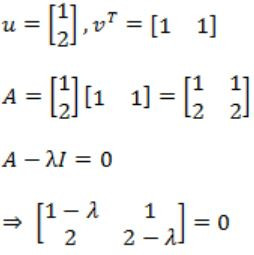⇒ (1 - λ)(2 - λ) - 2 = 0
⇒ λ2 - 3λ=0
λ = 0, 3
So maximum is 3.
 Question 12

The postorder traversal of a binary tree is 8, 9, 6, 7, 4, 5, 2, 3, 1. The inorder traversal of the same tree is 8, 6, 9, 4, 7, 2, 5, 1, 3. The height of a tree is the length of the longest path from the root to any leaf. The height of the binary tree above is _______.

 A 1 B 2 C 3 D 4
Data-Structures       Trees
Question 12 Explanation:
Post – 8 9 6 7 4 5 2 3 1
In – 8 6 9 4 7 2 5 1 3
Post: 8 9 6 7 4 5 2 3 1→(root)
In: 8 6 9 4 7 2 5→(left subtree) 13→(right subtree)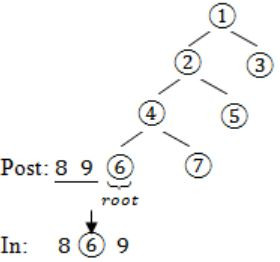Question 13

Two people, P and Q, decide to independently roll two identical dice, each with 6 faces, numbered 1 to 6. The person with the lower number wins. In case of a tie, they roll the dice repeatedly until there is no tie. Define a trial as a throw of the dice by P and Q. Assume that all 6 numbers on each dice are equi-probable and that all trials are independent. The probability (rounded to 3 decimal places) that one of them wins on the third trial is __________.

 A 0.021 B 0.022 C 0.023 D 0.024
Engineering-Mathematics       Probability
Question 13 Explanation:
When two identical dice are rolled
⇾ A person wins who gets lower number compared to other person.
⇾ There could be “tie”, if they get same number.
Favorable cases = {(1, 1), (2, 2), (3, 3), (4, 4), (5, 5), (6, 6)}
Probability (tie) = 6/36 (when two dice are thrown, sample space = 6 × 6 = 36)
= 1/6
“Find the probability that one of them wins in the third attempt"
⇾ Which means, first & second time it should be tie and third time it should not be tie
⇾ P (tie) * P (tie) * P (not tie)
⇒ 1/6* 1/6 * (1 - 1/6)
⇒ (5/36×6)
= 0.138/6
= 0.023
 Question 14

In an Entity-Relationship (ER) model, suppose R is a many-to-one relationship from entity set E1 to entity set E2. Assume that E1 and E2 participate totally in R and that the cardinality of E1 is greater than the cardinality of E2.

Which one of the following is true about R?

 A Every entity in E1 is associated with exactly one entity in E2. B Some entity in E1 is associated with more than one entity in E2. C Every entity in E2 is associated with exactly one entity in E1. D Every entity in E2 is associated with at most one entity in E1.
Database-Management-System       ER-Model
Question 14 Explanation:The M : 1 relationship holds between two entities E1 and E2, in which each tuple from E2 is in relation with many tuples of E1. One tuple from E1 is in relation with only one tuple of E2. It is given that participation from both the sides is total and the cardinality of E1 is greater than E2.Therefore, every entity E1 is associated with exactly one entity in E2.
 Question 15

Consider a process executing on an operating system that uses demand paging. The average time for a memory access in the system is M units if the corresponding memory page is available in memory and D units if the memory access causes a page fault. It has been experimentally measured that the average time taken for a memory access in the process is X units.

Which one of the following is the correct expression for the page fault rate experienced by the process?

 A (D-M)/(X-M) B (X-M)/(D-M) C (D-X)/(D-M) D (X-M)/(D-X)
Operating-Systems       Virtual Memory
Question 15 Explanation:
Let ‘P’ be page fault rate then, average memory access time
X = (1 - P)M + D × P
X = M ∙ PM + DP
(X - M) = P(D - M)
⇒ P = (X - M) / (D - M)
 Question 16

Consider a long-lived TCP session with an end-to-end bandwidth of 1 Gbps (= 109 bits/second). The session starts with a sequence number of 1234. The minimum time (in seconds, rounded to the closest integer) before this sequence number can be used again is _________.

 A 33 B 34 C 35 D 36
Computer-Networks       TCP
Question 16 Explanation:
In TCP, Sequence number field is 32 bit, which means 232 sequence number per byte are possible. Whatever be the starting sequence number the possible number will be 232bytes
The process of using all the sequence number and repeating a previously used sequence number.
The time taken to wrap around is called wrap around time:
Minimum Time = Wrap around time = Total number of bits in sequence number / Bandwidth = 232 * 8 / 109 = 34.35 == 34 (closest integer)
 Question 17

A 32-bit wide main memory unit with a capacity of 1 GB is built using 256M × 4-bit DRAM chips. The number of rows of memory cells in the DRAM chip is 214. The time taken to perform one refresh operation is 50 nanoseconds. The refresh period is 2 milliseconds. The percentage (rounded to the closest integer) of the time available for performing the memory read/write operations in the main memory unit is _________.

 A 59% B 60% C 61% D 62%
Computer-Organization       Memory-Interfacing
Question 17 Explanation:
Time taken to refresh one row = 50 ns
There are 214 rows, so time taken to refresh all the rows = 214 * 50ns = 0.82 milliseconds
It is given that total refresh period is 2ms. The refresh period contains the time to refresh all the rows and also the time to perform read/write operation.
So % time spent in refresh = (Time taken to refresh all rows / refresh period)*100
= (0.82 ms / 2ms)*100
= 41%
So the % of time for read/write operation = 100 - 41 = 59%
 Question 18

The chromatic number of the following graph is _______.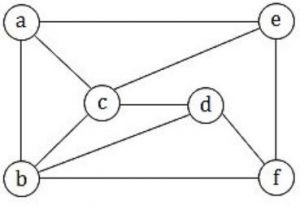A 1 B 2 C 3 D 4
Engineering-Mathematics       Graph-Theory
Question 18 Explanation:
Chromatic number of the following graph is “3”Question 19

Match the following:

```           Field                       Length in bits
P. UDP Header's Port Number               I.  48
Q. Ethernet MAC Address                   II.  8
R. IPv6 Next Header                       III.32
S. TCP Header's Sequence Number           IV. 16
```
 A P-III, Q-IV, R-II, S-I B P-II, Q-I, R-IV, S-III C P-IV, Q-I, R-II, S-III D P-IV, Q-I, R-III, S-II
Computer-Networks       Header
Question 19 Explanation:
P. UDP Header’s Port Number - 16 bits
Q. Ethernet MAC Address - 48 bits
R. IPV6 Next Header - 8 bits
S. TCP Header’s Sequence Number - 32 bits
 Question 20

A queue is implemented using a non-circular singly linked list. The queue has a head pointer and a tail pointer, as shown in the figure. Let n denote the number of nodes in the queue. Let 'enqueue' be implemented by inserting a new node at the head, and 'dequeue' be implemented by deletion of a node from the tail.Which one of the following is the time complexity of the most time-efficient implementation of 'enqueue' and 'dequeue, respectively, for this data structure?

 A θ(1), θ(1) B θ(1), θ(n) C θ(n), θ(1) D θ(n), θ(n)
Data-Structures       Queues-and-Linked-Lists
Question 20 Explanation:
For insertion of node at the beginning of linked list only need manipulation of pointers so θ(1) time.
But if we have pointer to the tail of the list in order to delete it, we need the address of the 2nd last node which can be obtained by traversing the list which takes O(n) time.
 Question 21

Consider a system with 3 processes that share 4 instances of the same resource type. Each process can request a maximum of K instances. Resource instances can be requested and released only one at a time. The largest value of K that will always avoid deadlock is _________.

 A 2 B 3 C 4 D 5
Operating-Systems       Deadlock
Question 21 Explanation:
No. of process = 3
No. of resources = 4
Let’s distribute each process one less than maximum demands i.e., (k-1) resources.
So, for three processes, 3(k – 1) resources.
For deadlock avoidance provide an additional resource to any one of the process.
∴ Total resources required to avoid deadlock in any case is 3(k – 1) + 1 = 3k – 2
Now this 3k – 2 should be less than equal to available no. of resources, i.e.,
3k – 2 ≤ 4
k ≤ 2
So maximum value of k = 2
 Question 22

Consider the following statements regarding the slow start phase of the TCP congestion control algorithm. Note that cwnd stands for the TCP congestion window and MSS denotes the Maximum Segment Size.

(i) The cwnd increase by 2 MSS on every successful acknowledgement.
(ii) The cwnd approximately doubles on every successful acknowledgedment.
(iii) The cwnd increase by 1 MSS every round trip time.
(iv) The cwnd approximately doubles every round trip time.

Which one of the following is correct?

 A Only (ii) and (iii) are true B Only (i) and (iii) are true C Only (iv) is true D Only (i) and (iv) are true
Computer-Networks       TCP-Congestion-Window
Question 22 Explanation:
In Slow-start, the value of the Congestion Window will be increased by 1 MSS with each acknowledgement (ACK) received, and effectively doubling the window size each round-trip time
Initially, TCP starts with cwnd of 1 MSS. On every ack, it increases cwnd by 1 MSS.
That is, cwnd doubles every RTT.
Initially sends 1 segment. On ack, sends 2 segments.
After these 2 acks come back, sends 4 segments etc.
TCP rate increases exponentially during slow start.
Slow start continues till cwnd reaches threshold.
After threshold is reached, cwnd increases more slowly, by one 1 MSS every RTT.
 Question 23

Which one of the following statements is FALSE?

 A Context-free grammar can be used to specify both lexical and syntax rules. B Type checking is done before parsing. C High-level language programs can be translated to different Intermediate Representations. D Arguments to a function can be passed using the program stack.
Compiler-Design       Compilers
Question 23 Explanation:
Type checking is done in semantic analysis phase after syntax analysis phase (i.e., after parsing).
 Question 24

Let N be an NFA with n states. Let k be the number of states of a minimal DFA which is equivalent to N. Which one of the following is necessarily true?

 A k ≥ 2n B k ≥ n C k ≤ n2 D k ≤ 2n
Theory-of-Computation       Finite-Automata
Question 24 Explanation:
The number of states in DFA is always less than equal to 2no. of states in NFA.
In other words, if number of states in NFA is “n” then the corresponding DFA have at most 2n states.
Hence k ≤ 2n is necessarily true.
 Question 25

The following are some events that occur after a device controller issues an interrupt while process L is under execution.

(P) The processor pushes the process status of L onto the control stack.
(Q) The processor finishes the execution of the current instruction.
(R) The processor executes the interrupt service routine.
(S) The processor pops the process status of L from the control stack.
(T) The processor loads the new PC value based on the interrupt.
 A QPTRS B PTRSQ C TRPQS D QTPRS
Computer-Organization       Interrupt-Service-Routing
Question 25 Explanation:
The sequence of execution in the case of an interrupt while executing a process L is:
(Q) The processor finishes the execution of the current instruction
(P) The processor pushes the process status of L onto the control stack
(T) The processor loads the new PC value based on the interrupt
(R) The processor executes the interrupt service routine
(S) The processor pops the process status of L from the control stack
This is the sequence because when a process is in the middle of execution if an interrupt comes then that process execution is completed then the interrupt is serviced.
 Question 26

Let ⊕ and ⊙ denote the Exclusive OR and Exclusive NOR operations, respectively. Which one of the following is NOT CORRECT?

 ABCDDigital-Logic-Design       Logic-Gates
Question 26 Explanation:Question 27

Let G be a finite group on 84 elements. The size of a largest possible proper subgroup of G is _________.

 A 41 B 42 C 43 D 44
Engineering-Mathematics       Set-Theory
Question 27 Explanation:
Lagranges Theorem:
For any group ‘G’ with order ‘n’, every subgroup ‘H’ has order ‘k’ such that ‘n’ is divisible by ‘k’.
Solution:
Given order n = 84
Then the order of subgroups = {1, 2, 3, 4, 6, 7, 12, 14, 21, 28, 42, 84}
As per the proper subgroup definition, it should be “42”.
 Question 28

Which one of the following is a closed form expression for the generating function of the sequence {an}, where an = 2n+3 for all n = 0, 1, 2, …?

 A 3/(1-x)2 B 3x/(1-x)2 C 2-x/(1-x)2 D 3-x/(1-x)2
Engineering-Mathematics       Combinatorics
Question 28 Explanation:Question 29

The set of all recursively enumerable languages is

 A closed under complementation. B closed under intersection. C a subset of the set of all recursive languages. D an uncountable set.
Theory-of-Computation       Closure-Property
Question 29 Explanation:
Recursive enumerable languages are closed under intersection.
Recursive enumerable languages are not closed under Complementation.
Recursive enumerable languages are a countable set, as every recursive enumerable language has a corresponding Turing Machine and set of all Turing Machine is countable.
Recursive languages are subset of recursive enumerable languages.
 Question 30

Consider the sequential circuit shown in the figure, where both flip-flops used are positive edge-triggered D flip-flops.The number of states in the state transition diagram of this circuit that have a transition back to the same state on some value of "in" is ______

 A 2 B 3 C 4 D 5
Digital-Logic-Design       Sequential-Circuits
Question 30 Explanation:
Let,Now lets draw characteristic table,
D1 = Q0
D0 = inQuestion 31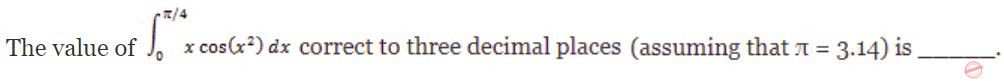A 0.289 B 0.298 C 0.28 D 0.29
Engineering-Mathematics       Calculus
Question 31 Explanation: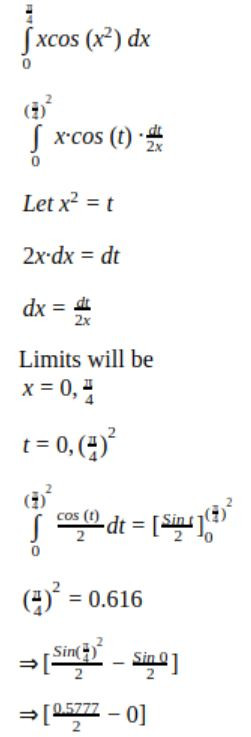Question 32

Consider the following two tables and four queries in SQL.

Book (isbn, bname), Stock (isbn, copies)
```Query 1:    SELECT B.isbn, S.copies
FROM Book B INNER JOIN Stock S
ON B.isbn = S.isbn;

Query 2:    SELECT B.isbn, S.copies
FROM Book B LEFT OUTER JOIN Stock S
ON B.isbn = S.isbn;

Query 3:    SELECT B.isbn, S.copies
FROM Book B RIGHT OUTER JOIN Stock S
ON B.isbn = S.isbn;

Query 4:    SELECT B.isbn, S.copies
FROM Book B FULL OUTER JOIN Stock S
ON B.isbn = S.isbn;
```

Which one of the queries above is certain to have an output that is a superset of the outputs of the other three queries?

 A Query 1 B Query 2 C Query 3 D Query 4
Database-Management-System       SQL
Question 32 Explanation:
Given two tables are,
Book (isbn, bname)
Stock (isbn, copies)
isbn is a primary key of Book and isbn is a foreign key of stock referring to Book table.
For example:Query 1:
INNER JOIN keyword selects records that have matching values in both tables (Book and Stock).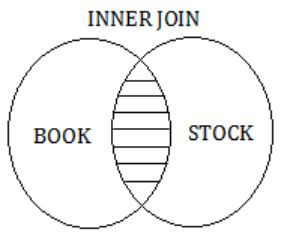So, the result of Query 1 is,Query 2:
The LEFT OUTER JOIN keyword returns all records from the left table (Book) and the matched records from the right table (Stock).
The result is NULL from the right side, if there is no match.So, the result of Query 2 is,Query 3:
The RIGHT OUTER JOIN keyword returns all records from the right table (Stock), and the matched records from the left table(BOOK).
The result is NULL from the left side, when there is no match.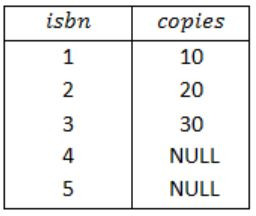Query 4:
The FULL OUTER JOIN keyword return all records when there is a match in either left (Book) or right (Stock) table records.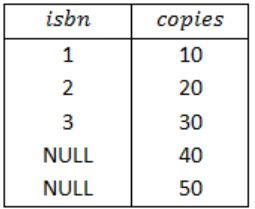So, the result of Query 4 is,Therefore, from the result of above four queries, a superset of the outputs of the Query 1, Query 2 and Query 3 is Query 4.
Note:
If we take isbn as a primary key in both the tables Book and Stock and foreign key, in one of the tables then also will get option (D) as the answer.
 Question 33

Consider the following C program:

```#include <stdio.h>

int counter = 0;

int calc (int a, int b) {
int c;

counter++;
if (b==3) return (a*a*a) ;
else  {
c = calc (a, b/3) ;
return (c*c*c) ;
}
}

int main ()  {
calc (4, 81);
printf ("%d", counter) ;
}
```

The output of this program is ______.

 A 4 B 5 C 6 D 7
Programming       Programming
Question 33 Explanation:Question 34

Consider the following processor design characteristics.

I. Register-to-register arithmetic operations only
II. Fixed-length instruction format
III. Hardwired control unit

Which of the characteristics above are used in the design of a RISC processor?

 A I and II only B II and III only C I and III only D I, II and III
Computer-Organization       RISC-and-CISC
Question 34 Explanation:
RISC processor has all the three given characteristics.
So option D is the correct answer.
 Question 35

Consider the following C program.

```#include <stdio.h>
struct Outnode {
char x, y, z ;
};

int main () {
struct Ournode p = {'1', '0', 'a'+2} ;
struct Ournode *q = &p ;
printf ("%c, %c", *((char*)q+1), *((char*)q+2)) ;
return 0 ;
}
```

The output of this program is

 A 0, c B 0, a+2 C ‘0’, ‘a+2’ D ‘0’, ‘c’
Programming       Programming
Question 35 Explanation:
char x = ‘a’+2;
The x variable here stores a character ‘c’ in it.
Because +2 will increment ascii value of a from 92 to 95.
Hence the structure p contains 3 character values and they are ‘1’, ‘0’, and ‘c’.
q is a pointer pointing to structure p.
Hence q is pointing to ‘1’, q+1 pointing to ‘0’ and q+2 pointing to ‘c’.
Option d cannot be correct, as though they are characters, printf will not print them in single quotes.
 Question 36

The size of the physical address space of a processor is 2P bytes. The word length is 2W bytes. The capacity of cache memory is 2N bytes. The size of each cache block is 2M words. For a K-way set-associative cache memory, the length (in number of bits) of the tag field is

 A P - N - log2K B P - N + log2K C P - N - M - W - log2K D P - N - M - W + log2K
Computer-Organization       Cache
Question 36 Explanation:
Physical memory is of size 2P bytes.
Each word is of size 2W bytes.
Number of words in physical memory = 2(P-W)
So the physical address is P-W bits
Cache size is 2N bytes.
Number of words in the cache = 2(N-W)
Block size is 2M words
No. of blocks in the cache = 2(N-W-M)
Since it is k-way set associative cache, each set in the cache will have k blocks.
No. of sets = 2(N-W-M ) / k
SET bits = N-W-M-logk
Block offset = M
TAG bits = P-W-(N-M-W-logk)-M = P-W-N+M+W+logk-M = P - N + logk
 Question 37

Consider the following C program:

```#include<stdio.h>

void fun1(char *s1, char *s2)  {
char *tmp;
tmp = s1;
s1 = s2;
s2 = tmp;
}

void fun2(char **s2, char **s2)  {
char *tmp;
tmp = *s1;
*s1 = *s2;
*s2 = tmp;
}

int main ()  {
char *str1 = "Hi", *str2 = "Bye";
fun1(str1, str2);     printf("%s %s", str1, str2);
fun2(&str1, &str2);   printf("%s %s", str1, str2);
return 0;
}
```

The output of the program above is

 A Hi Bye Bye Hi B Hi Bye Hi Bye C Bye Hi Hi Bye D Bye Hi Bye Hi
Programming       Programming
Question 37 Explanation:
The first call to the function ‘func1(str1, str2);’ is call by value.
Hence, any change in the formal parameters are NOT reflected in actual parameters.
Hence, str1 points at “hi” and str2 points at “bye”.
The second call to the function ‘func2(&str1, &str2);’ is call by reference.
Hence, any change in formal parameters are reflected in actual parameters.
Hence, str1 now points at “bye” and str2 points at “hi”.
Hence answer is “hi bye bye hi”.
 Question 38

Consider the following undirected graph G:Choose a value for x that will maximize the number of minimum weight spanning trees (MWSTs) of G. The number of MWSTs of G for this value of x is _________.

 A 4 B 5 C 6 D 7
Algorithms       Minimum-Spanning-Tree
Question 38 Explanation:
Here, x = 5 because it is having maximum number of spanning trees.
If x = 5 then the total number of MWSTs are 4.
If r = 1If r = 2If r = 3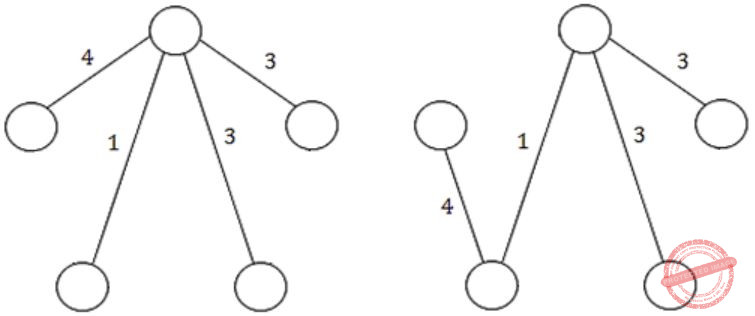If r = 4If r = 5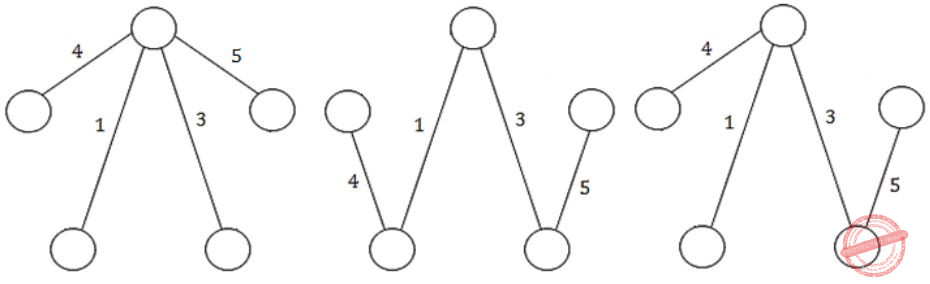Question 39

A processor has 16 integer registers (R0, R1, …, R15) and 64 floating point registers (F0, F1, …, F63). It uses a 2-byte instruction format. There are four categories of instructions: Type-1, Type-2, Type-3 and Type-4. Type-1 category consists of four instructions, each with 3 integer register operands (3Rs). Type-2 category consists of eight instructions, each with 2 floating point register operands (2Fs). Type-3 category consists of fourteen instructions, each with one integer register operand and one floating point register operand (1R+1F). Type-4 category consists of N instructions, each with a floating point register operand (1F).

The maximum value of N is ________.

 A 32 B 33 C 34 D 35
Computer-Organization       Machine-Instructions
Question 39 Explanation:
Instruction size = 2−byte.
So, total number of instruction encodings = 216
There are 16 possible integer registers, so no. of bits required for an integer operand = 4
There are 64 possible floating point registers, so no. of bits required for a floating point operand = 6
Type-1 instructions:
There are 4 type-1 instructions and each takes 3 integer operands.
No. of encodings consumed by type-1 = 4 × 24 × 24 × 24 = 214.
Type-2 instructions:
There are 8 type-2 instructions and each takes 2 floating point operands.
No. of encodings consumed by Type-2 instructions = 8 × 26 x 26 = 215.
Type-3 instructions:
There are 14 type-3 instructions and each takes one integer operand and one floating point operand.
No. of encodings consumed by Type-3 instructions = 14 × 24 × 26 = 14336.
So, no. of encodings left for Type-4 = 216 − (214 + 215 + 14336) = 2048.
Since type-4 instructions take one floating point register, no. of different instructions of Type-4 = 2048 / 64 = 32.
 Question 40

Assume that multiplying a matrix G1 of dimension p×q with another matrix G2 of dimension q×r requires pqr scalar multiplications. Computing the product of n matrices G1G2G3…Gn can be done by parenthesizing in different ways. Define GiGi+1 as an explicitly computed pair for a given parenthesization if they are directly multiplied. For example, in the matrix multiplication chain G1G2G3G4G5G6 using parenthesization(G1(G2G3))(G4(G5G6)), G2G3 and G5G6 are the only explicitly computed pairs.

Consider a matrix multiplication chain F1F2F3F4F5, where matrices F1, F2, F3, F4 and F5 are of dimensions 2×25, 25×3, 3×16, 16×1 and 1×1000, respectively. In the parenthesization of F1F2F3F4F5 that minimizes the total number of scalar multiplications, the explicitly computed pairs is/ are

 A F1F2 and F3F4 only B F2F3 only C F3F4 only D F1F2 and F4F5 only
Engineering-Mathematics       Linear-Algebra
Question 40 Explanation:
→ As per above information, the total number of scalar multiplications are 2173.
→ Optimal Parenthesization is:
((F1(F2(F3 F4)))F5)
→ But according to the problem statement we are only considering F3, F4 explicitly computed pairs.
 Question 41

Consider the following parse tree for the expression a#b\$c\$d#e#f, involving two binary operators \$ and #.Which one of the following is correct for the given parse tree?

 A \$ has higher precedence and is left associative; # is right associative B # has higher precedence and is left associative; \$ is right associative C \$ has higher precedence and is left associative; # is left associative D # has higher precedence and is right associative; \$ is left associative
Compiler-Design       Associativity-and-Precedence
Question 41 Explanation:
Since \$ will be evaluated before # so \$ has higher precedence and the left \$ i.e., in b\$c\$d the left “\$” (i.e., b\$c) will be evaluated first so it is left associative, whereas # is right associative (as in d#e#f) , the right one (i.e., e#f) will be evaluated first.
 Question 42

Consider the following solution to the producer-consumer synchronization problem. The shared buffer size is N. Three semaphores empty, full and mutex are defined with respective initial values of 0, N and 1. Semaphore empty denotes the number of available slots in the buffer, for the consumer to read from. Semaphore full denotes the number of available slots in the buffer, for the producer to write to. The placeholder variables, denoted by P, Q, R and S, in the code below can be assigned either empty or full. The valid semaphore operations are: wait() and sigmal().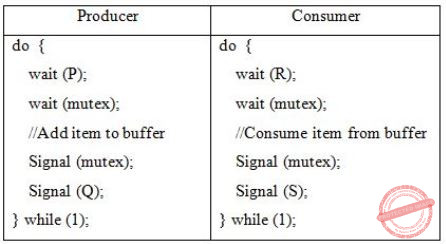Which one of the following assignments to P, Q, R and S will yield the correct solution?

 A P: full, Q: full, R: empty, S: empty B P: empty, Q: empty, R: full, S: full C P: full, Q: empty, R: empty, S: full D P: empty, Q: full, R: full, S: empty
Operating-Systems       Process-Synchronization
Question 42 Explanation:
P=full, Q=empty, R=empty, S=full
Initial: mutex = 1
empty = 0
full = N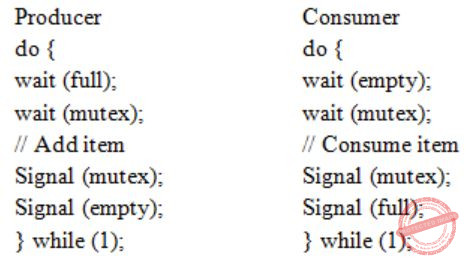Question 43

Consider the first-order logic sentence

φ ≡ ∃s∃t∃u∀v∀w∀x∀y ψ(s,t,u,v,w,x,y)

where ψ(s,t,u,v,w,x,y) is a quantifier-free first-order logic formula using only predicate symbols, and possibly equality, but no function symbols. Suppose φ has a model with a universe containing 7 elements.

Which one of the following statements is necessarily true?

 A There exists at least one model of φ with universe of size less than or equal to 3. B There exists no model of φ with universe of size less than or equal to 3. C There exists no model of φ with universe of size greater than 7. D Every model of φ has a universe of size equal to 7.
Engineering-Mathematics       Propositional-Logic
Question 43 Explanation:
φ = ∃s∃t∃u∀v∀w∀x∀y ψ (s,t,u,v,w,x,y)
"∃" there exists quantifier decides whether a sentence belong to the model or not.
i.e., ~∃ will make it not belong to the model. (1) We have ‘7’ elements in the universe, So max. size of universe in a model = ‘7’
(2) There are three '∃' quantifiers, which makes that a model have atleast “3” elements. So, min. size of universe in model = ‘7’.
(A) is False because: (2)
(B) is true
(C) is false because of (1)
(D) is false, because these all models with size {3 to 7} not only ‘7’.
 Question 44

Let G be a simple undirected graph. Let TD be a depth first search tree of G. Let TB be a breadth first search tree of G. Consider the following statements.

(I) No edge of G is a cross edge with respect to TD.
(A cross edge in G is between two nodes neither of which is an ancestor of the other in TD.)
(II) For every edge (u,v) of G, if u is at depth i and v is at depth j in TB, then ∣i−j∣ = 1.

Which of the statements above must necessarily be true?

 A I only B II only C Both I and II D Neither I nor II
Data-Structures       Graphs
Question 44 Explanation:
I. Undirected graph do not have cross edges in DFS. But can have cross edges in directed graph. Hence True.
II. Just draw a triangle ABC. Source is A. Vertex B and C are at same level at distance 1.
There is an edge between B and C too. So here |i - j| = |1 - 1| = 0. Hence, False.
 Question 45

Consider the following C code. Assume that unsigned long int type length is 64 bits.

```        unsigned long int fun(unsigned long int n)  {
unsigned long int i, j, j=0, sum = 0;
for (i = n; i > 1; i = i/2) j++;
for ( ; j > 1; j = j/2) sum++;
return sum;
}
```

The value returned when we call fun with the input 240 is

 A 4 B 5 C 6 D 40
Programming       Programming
Question 45 Explanation:
Since 240 is the input, so first loop will make j = 40.
Next for loop will divide j value (which is 40) by 2, each time until j>1.
j loop starts:
j=40 & sum=1
j=20 & sum=2
j=10 & sum=3
j=5 & sum=4
j=2 & sum=5
j=1 & break
So, sum = 5.
 Question 46

Consider a storage disk with 4 platters (numbered as 0, 1, 2 and 3), 200 cylinders (numbered as 0, 1, … , 199), and 256 sectors per track (numbered as 0, 1, … 255). The following 6 disk requests of the form [sector number, cylinder number, platter number] are received by the disk controller at the same time:

[120, 72, 2], [180, 134, 1], [60, 20, 0], [212, 86, 3], [56, 116, 2], [118, 16, 1]

Currently head is positioned at sector number 100 of cylinder 80, and is moving towards higher cylinder numbers. The average power dissipation in moving the head over 100 cylinders is 20 milliwatts and for reversing the direction of the head movement once is 15 milliwatts. Power dissipation associated with rotational latency and switching of head between different platters is negligible.

The total power consumption in milliwatts to satisfy all of the above disk requests using the Shortest Seek Time First disk scheduling algorithm is ______ .

 A 85 B 86 C 87 D 88
Operating-Systems       Disk-Scheduling
Question 46 Explanation:
From the above 36 no. question we are getting
→ A storage disk - 4 platters(0, 1, 2 & 3), Cylinder - 200 (0, 1, …, 199) , 256 sector per track.
In the above question the disk requests are given in the form of 〈sector no, cylinder no, platter no〉.
In SSTF (Shortest Seek Time First) Disk Scheduling algorithm, requests having shortest seek time are executed first.
So, the seek time of every request is calculated in advance in queue and then they are scheduled according to their calculated seek time. Head is positioned at 80 and moving towards higher cylinder no.Head Movement in SSTF = (86 – 80) + (86 – 72) + (134 – 72) + (134 – 16) = 200
P1: Power dissipated by 200 movements = 0.2 * 200 = 40 mW
Power dissipated in reversing Head direction once = 15 mW
No. of times Head changes its direction = 3 P2: Power dissipated in reversing Head direction = 3 * 15 = 45 mW
Total power consumption is P1 + P2 = 85 mW
 Question 47

Consider the unsigned 8-bit fixed point binary number representation below,

b7b6b5b4b3 ⋅ b2b1b0

where the position of the binary point is between b3 and b2 . Assume b7 is the most significant bit. Some of the decimal numbers listed below cannot be represented exactly in the above representation:

(i) 31.500    (ii) 0.875    (iii) 12.100    (iv) 3.001

Which one of the following statements is true?

 A None of (i), (ii), (iii), (iv) can be exactly represented B Only (ii) cannot be exactly represented C Only (iii) and (iv) cannot be exactly represented D Only (i) and (ii) cannot be exactly represented
Digital-Logic-Design       Number-Systems
Question 47 Explanation:
(i) (31.5)10 = (11111.100)2 = 24 + 23 + 22 + 21 + 20 + 2-1
= 16 + 8 + 4 + 2 + 1 + 0.5
= (31.5)10
(ii) (0.875)10 = (00000.111)2
= 2-1 + 2-2 + 2-3
= 0.5 + 0.25 + 0.125
= (0.875)10
(iii) (12.100)10
It is not possible to represent (12.100)10
(iv) (3.001)10 It is not possible to represent (3.001)10
 Question 48

In a system, there are three types of resources: E, F and G. Four processes P0, P1, P2 and P3 execute concurrently. At the outset, the processes have declared their maximum resource requirements using a matrix named Max as given below. For example, Max[P2, F] is the maximum number of instances of F that P2 would require. The number of instances of the resources allocated to the various processes at any given state is given by a matrix named Allocation.

Consider a state of the system with the Allocation matrix as shown below, and in which 3 instances of E and 3 instances of F are the only resources available.From the perspective of deadlock avoidance, which one of the following is true?

 A The system is in safe state. B The system is not in safe state, but would be safe if one more instance of E were available. C The system is not in safe state, but would be safe if one more instance of F were available. D The system is not in safe state, but would be safe if one more instance of G were available.
Operating-Systems       Deadlock
Question 48 Explanation:
〈E, F, G〉 = 〈3, 3, 0〉Safe sequence:
〈P0, P2, P1, P3
P0: P0 can be allotted 〈3,3,0〉.
After completion Available = 〈4,3,1〉
P2: P2 can be allotted 〈0,3,0〉.
After completion: Available = 〈5,3,4〉
P1: P1 can be allotted 〈1,0,2〉.
After completion: Available = 〈6,4,6〉
P3: P3 can be allotted 〈3,4,1〉.
After completion: Available = 〈8,4,6〉
 Question 49

Consider the following four relational schemas. For each schema, all non-trivial functional dependencies are listed. The underlined attributes are the respective primary keys.

```Schema I: Registration(rollno, courses)
Field ‘courses’ is a set-valued attribute containing the set of
courses a student has registered for.
Non-trivial functional dependency
rollno → courses

Schema II: Registration (rollno, coursid, email)
Non-trivial functional dependencies:
rollno, courseid → email
email → rollno

Schema III: Registration (rollno, courseid, marks, grade)
Non-trivial functional dependencies:
rollno, courseid, → marks, grade
marks → grade

Schema IV: Registration (rollno, courseid, credit)
Non-trivial functional dependencies:
rollno, courseid → credit
courseid → credit
```

Which one of the relational schemas above is in 3NF but not in BCNF?

 A Schema I B Schema II C Schema III D Schema IV
Database-Management-System       Normalization
Question 49 Explanation:
Schema I:
Registration (rollno, courses) rollno → courses
For the given schema Registration ‘rollno’ is a primary key.
Left-side of the functional dependency is a superkey so, Registration is in BCNF.
Schema II:
Registrstion (rollno, courseid, email)
rollno, courseid → email
email → rollno
From the given schema the candidate key is (rollno + courseid).
There is no part of the key in the left hand of the FD’s so, it is in 2NF.
In the FD email→rollno, email is non-prime attribute but rollno is a prime attribute.
So, it is not a transitive dependency.
No transitive dependencies so, the schema is in 3NF.
But in the second FD email→rollno, email is not a superkey.
So, it is violating BCNF.
Hence, the schema Registration is in 3NF but not in BCNF.
Schema III:
Registration (rollno, courseid, marks, grade)
rollno, courseid → marks, grade
marks → grade
For the schema the candidate key is (rollno + courseid).
There are no part of the keys are determining non-prime attributes.
So, the schema is in 2NF.
In the FD marks → grade, both the attributes marks and grade are non-prime.
So, it is a transitive dependency.
The FD is violating 3NF.
The schema Registration is in 2NF but not in 3NF.
Schema IV:
Registration (rollno, courseid, credit)
rollno, courseid → credit
courseid → credit
The candidate key is (rollno + courseid).
In the FD, courseid → credit, courseid is part of the key (prime attribute) and credit is non-prime.
So, it is a partial dependency.
The schema is violating 2NF.
 Question 50

Given a language L, define Li as follows:

L0 = {ε}
Li = Li-1∙L for all i>0

The order of a language L is defined as the smallest k such that Lk = Lk+1.

Consider the language L1 (over alphabet 0) accepted by the following automaton.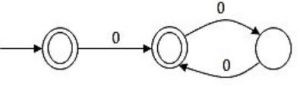The order of L1 is ______.

 A 2 B 3 C 4 D 5
Theory-of-Computation       Finite-Automata
Question 50 Explanation:
The regular expression for L1 : ϵ + 0 (00)*
Now L10 = ϵ and L11 = ϵ . (ϵ+0 (00)*) = ϵ + 0 (00)* = L1
Now L12 = L11 .
L1 = L1 .
L1 = (ϵ + 0 (00)*) (ϵ + 0 (00)*)
= (ϵ + 0 (00)* + 0(00)* + 0(00)*0(00)*)
= (ϵ + 0 (00)* + 0(00)*0(00)* ) = 0*
As it will contain epsilon + odd number of zero + even number of zero, hence it is 0*
Now L13 = L12 .
L1 = 0* (ϵ + 0 (00)*) = 0* + 0*0(00)* = 0*
Hence L12 = L13
Or L12 = L12+1 ,
hence the smallest k value is 2.
 Question 51

Consider a simple communication system where multiple nodes are connected by a shared broadcast medium (like Ethernet or wireless). The nodes in the system use the following carrier-sense based medium access protocol. A node that receives a packet to transmit will carrier-sense the medium for 5 units of time. If the node does not detect any other transmission in this duration, it starts transmitting its packet in the next time unit. If the node detects another transmission, it waits until this other transmission finishes, and then begins to carrier-sense for 5 time units again. Once they start to transmit, nodes do not perform any collision detection and continue transmission even if a collision occurs. All transmissions last for 20 units of time. Assume that the transmission signal travels at the speed of 10 meters per unit time in the medium.

Assume that the system has two nodes P and Q, located at a distance d meters from each other. P starts transmitting a packet at time t=0 after successfully completing its carrier-sense phase. Node Q has a packet to transmit at time t=0 and begins to carrier-sense the medium.

The maximum distance d (in meters, rounded to the closest integer) that allows Q to successfully avoid a collision between its proposed transmission and P’s ongoing transmission is ___________.

 A 50 B 51 C 52 D 53
Computer-Networks       Ethernet
Question 51 Explanation:
Node senses the medium for 5 unit time. it means, any packet which arrives within 5 unit will be sensed and keep the channel busy.
Now signal travels at the speed of 10 meters per unit time.
Therefore, in 5 unit time, it can travel a maximum distance (d) of 50 m (5*10), which allows the receiver (Q) to sense that the channel is busy.
 Question 52

A lexical analyzer uses the following patterns to recognize three tokens T1, T2, and T3 over the alphabet {a,b,c}.

T1: a?(b∣c)*a
T2: b?(a∣c)*b
T3: c?(b∣a)*c

Note that ‘x?’ means 0 or 1 occurrence of the symbol x. Note also that the analyzer outputs the token that matches the longest possible prefix.

If the string bbaacabc is processes by the analyzer, which one of the following is the sequence of tokens it outputs?

 A T1T2T3 B T1T1T3 C T2T1T3 D T3T3
Compiler-Design       Compilers
Question 52 Explanation:
a? means either 0 or 1 occurrence of “a”, so we can write T1, T2 and T3 as:
T1 : (b+c)*a + a(b+c)*a
T2 : (a+c)*b + b(a+c)*b
T3 : (b+a)*c + c(b+a)*c
Now the string is: bbaacabc
Please NOTE:
Token that matches the longest possible prefix
We can observe that the longest possible prefix in string is : bbaac which can be generated by T3.
After prefix we left with “abc” which is again generated by T3 (as longest possible prefix).
So, answer is T3T3.
 Question 53

Consider Guwahati (G) and Delhi (D) whose temperatures can be classified as high (H), medium (M) and low (L). Let P(HG) denote the probability that Guwahati has high temperature. Similarly, P(MG) and P(LG) denotes the probability of Guwahati having medium and low temperatures respectively. Similarly, we use P(HD), P(MD) and P(LD) for Delhi.

The following table gives the conditional probabilities for Delhi’s temperature given Guwahati’s temperature.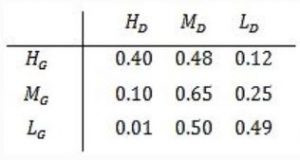Consider the first row in the table above. The first entry denotes that if Guwahati has high temperature (HG) then the probability of Delhi also having a high temperature (HD) is 0.40; i.e., P(HD ∣ HG) = 0.40. Similarly, the next two entries are P(MD ∣ HG) = 0.48 and P(LD ∣ HG) = 0.12. Similarly for the other rows.

If it is known that P(HG) = 0.2, P(MG) = 0.5, and P(LG) = 0.3, then the probability (correct to two decimal places) that Guwahati has high temperature given that Delhi has high temperature is _______ .

 A 0.6 B 0.61 C 0.62 D 0.63
Engineering-Mathematics       Linear-Algebra
Question 53 Explanation: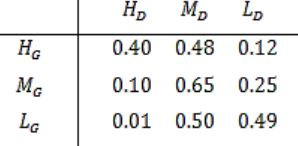The first entry denotes that if Guwahati has high temperature (HG ) then the probability that Delhi also having a high temperature (HD ) is 0.40.
P (HD / HG ) = 0.40
We need to find out the probability that Guwahati has high temperature.
Given that Delhi has high temperature (P(HG / HD )).P (HD / HG ) = P(HG ∩ HD ) / P(HD )
= 0.2×0.4 / 0.2×0.4+0.5×0.1+0.3×0.01
= 0.60
 Question 54

Consider the following program written in pseudo-code. Assume that x and y are integers.

```Count (x, y)  {
if (y != 1)  {
if (x != 1)  {
print("*") ;
Count (x/2, y) ;
}
else  {
y = y - 1 ;
Count (1024, y) ;
}
}
}
```

The number of times that the print statement is executed by the call Count(1024, 1024) is ______.

 A 10230 B 10231 C 10232 D 10233
Programming       PRogramming
Question 54 Explanation:
#include
int count=0;
Count(x,y){
if(y!=1){
if(x!=1){
printf("*");
count = count +1;
Count(x/2,y);
}
else{
y=y-1;
Count(1024,y);
}
}
}
void main()
{
Count(1024,1024);
printf("\n%d\n",count);
}Count ( ) is called recursively for every (y = 1023) & for every y, Count ( ) is called (x = 10) times = 1023 × 10 = 10230
 Question 55

Consider the following languages:

I. {ambncpdq ∣ m + p = n + q, where m, n, p, q ≥ 0}
II. {ambncpdq ∣ m = n and p = q, where m, n, p, q ≥ 0}
III. {ambncpdq ∣ m = n = p and p ≠ q, where m, n, p, q ≥ 0}
IV. {ambncpdq ∣ mn = p + q, where m, n, p, q ≥ 0}

Which of the above languages are context-free?

 A I and IV only B I and II only C II and III only D II and IV only
Theory-of-Computation       Context-Free-Language
Question 55 Explanation:
i) am bn cp dq | m+p = n+q,
m-n = q-p
First we will push a’s in the stack then we will pop a’s after watching b’s.
Then some of a’s might left in the stack.
Now we will push c’s in the stack and then pop c’s by watching d’s.
And then the remaining a’s will be popped off the stack by watching some more d’s.
And if no d’s is remaining and the stack is empty then the language is accepted by CFG.
ii) am bn cp dq | m=n, p=q
Push a’s in stack. Pop a’s watching b’s.
Now push c’s in stack.
Pop c’s watching d’s.
So it is context free language.
iii) am bn cp dq | m=n=p & p≠q
Here three variables are dependent on each other. So not context free.
iv) Not context free because comparison in stack can’t be done through multiplication operation.
 Question 56

Consider the following problems. L(G) denotes the language generated by a grammar G. L(M) denotes the language accepted by a machine M.

(I) For an unrestricted grammar G and a string w, whether w∈L(G)
(II) Given a Turing machine M, whether L(M) is regular
(III) Given two grammar G1 and G2, whether L(G1) = L(G2)
(IV) Given an NFA N, whether there is a deterministic PDA P such that N and P accept the same language

Which one of the following statement is correct?

 A Only I and II are undecidable B Only III is undecidable C Only II and IV are undecidable D Only I, II and III are undecidable
Theory-of-Computation       Decidability-and-Undecidability
Question 56 Explanation:
IV is trivial property, as every regular language is CFL also, so a language which has NFA must be regular and for every regular language we can have a deterministic PDA (as every regular language is DCFL).
I, II and III is undecidable.
 Question 57

Consider the relations r(A, B) and s(B, C), where s.B is a primary key and r.B is a foreign key referencing s.B. Consider the query

Q: r⋈(σB<5(s))

Let LOJ denote the natural left outer-join operation. Assume that r and s contain no null values.

Which one of the following is NOT equivalent to Q?

 A σB<5 (r ⨝ s) B σB<5 (r LOJ s) C r LOJ (σB<5(s)) D σB<5(r) LOJ s
Database-Management-System       Relational-Algebra
Question 57 Explanation:
Consider the following relations r(A, B) and S(B, C), where S.B is a primary key and r.B is a foreign key referencing S.B
Consider the following tables without NULL values.Q: r⨝(σB<5(S))
The result of σB<5(S) is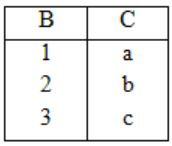The result of σB<5(S) isOption (A):
The result of r⨝S isThe result of σB<5(r⨝S) isOption (B):
The result of r LOJ S isThe result of σB<5(r LOJ S) isOption (C):
The result of σB<5(S) isNow, the result of r LOJ(σB<5(S))Option (D):
The result of σB<5(r) isNow, the result of σB<5(r) LOJ S isTherefore, from the output of above four options, the results of options, the results of options (A), (B) and (D) are equivalent to Q.
 Question 58

Consider an IP packet with a length of 4,500 bytes that includes a 20-byte IPv4 header and a 40-byte TCP header. The packet is forwarded to an IPv4 router that supports a Maximum Transmission Unit (MTU) of 600 bytes. Assume that the length of the IP header in all the outgoing fragments of this packet is 20 bytes. Assume that the fragmentation offset value stored in the first fragment is 0.

The fragmentation offset value stored in the third fragment is __________.

 A 144 B 145 C 146 D 147
Computer-Networks       IPv4-an-Fragmentation
Question 58 Explanation:
MTU = 600 bytes, IP header = 20 bytes
Therefore Payload = 600 - 20 = 580 bytes.
As we know fragment size should be multiple of 8 but 580 bytes is not a multiple of 8, therefore fragment size is 576 bytes.
Offset value of kth fragment = Fragment size *( kth fragment - 1) / scaling factor
Offset value of third fragment = 576 * (3-1) / 8 = 144
 Question 59

Let N be the set of natural numbers. Consider the following sets,

P: Set of Rational numbers (positive and negative)
Q: Set of functions from {0, 1} to N
R: Set of functions from N to {0, 1}
S: Set of finite subsets of N

Which of the above sets are countable?

 A Q and S only B P and S only C P and R only D P, Q and S only
Engineering-Mathematics       Linear-Algebra
Question 59 Explanation:
Set of rational numbers are countable. It is proved by various methods in literature.
Set of functions from {0,1} to N is countable as it has one to one correspondence to N.
Set of functions from N to {0,1} is uncountable, as it has one to one correspondence to set of real numbers between (0 and 1).
Set of finite subsets of N is countable.
 Question 60

Consider the weights and values of items listed below. Note that there is only one unit of each item.The task is to pick a subset of these items such that their total weight is no more than 11 Kgs and their total value is maximized. Moreover, no item may be split. The total value of items picked by an optimal algorithm is denoted by Vopt. A greedy algorithm sorts the items by their value-to-weight ratios in descending order and packs them greedily, starting from the first item in the ordered list. The total value of items picked by the greedy algorithm is denoted by Vgreedy.

The value of Vopt − Vgreedy is ______ .

 A 16 B 17 C 18 D 19
Algorithms       0/1-Knapsack-and-fractional-knapsack
Question 60 Explanation:
First sort value/weight in descending order as per the question:Vopt is clearly = 60
For Vgreedy use the table (Do not take the fraction as per the question),
Item 4 picked,
Profit = 24
Remaining weight = 11 – 2 = 9
Next item 3 picked (item 1 cannot be picked since its capacity is greater than available capacity),
Profit = 24 + 20 = 44
Remaining capacity = 9 – 4 = 5
Now no item can be picked with available capacity.
So Vgreedy = 44
∴ Vopt – Vgreedy = 60 – 44 = 16
 Question 61

Consider a matrix P whose only eigenvectors are the multiples of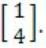Consider the following statements.

(I) P does not have an inverse
(II) P has a repeated eigenvalue
(III) P cannot be diagonalized

Which one of the following options is correct?

 A Only I and III are necessarily true B Only II is necessarily true C Only I and II are necessarily true D Only II and III are necessarily true
Engineering-Mathematics       Linear-Algebra
Question 61 Explanation:Though the multiple of a vector represents same vector, and each eigen vector has distinct eigen value, we can conclude that ‘p’ has repeated eigen value.
If the unique eigen value corresponds to an eigen vector e, but the repeated eigen value corresponds to an entire plane, then the matrix can be diagonalized, using ‘e’ together with any two vectors that lie in plane.
But, if all eigen values are repeated, then the matrix cannot be diagonalized unless it is already diagonal.
So (III) holds correct.
A diagonal matrix can have inverse, So (I) is false.
Then (II) and (III) are necessarily True.
 Question 62

The number of possible min-heaps containing each value from {1, 2, 3, 4, 5, 6, 7} exactly once is ___________.

 A 80 B 81 C 82 D 83
Data-Structures       Heap-Tree
Question 62 Explanation:
--> We have 7 distinct integers {1,2,3,4,5,6,7} and sort it
--> After sorting, pick the minimum element and make it the root of the min heap.
--> So, there is only 1 way to make the root of the min heap.
--> Now we are left with 6 elements.
--> Total ways to design a min heap from 6 elements = C(6,3) ∗ 2! ∗ C(3,3) ∗ 2! = 80

Note:
C(6,3)∗2! : Pick up any 3 elements for the left subtree and each left subtree combination can be permuted in 2! ways by interchanging the children and similarly, for right subtree .
 Question 63

Let G be a graph with 100! vertices, with each vertex labeled by a distinct permutation of the numbers 1, 2, …, 100. There is an edge between vertices u and v if and only if the label of u can be obtained by swapping two adjacent numbers in the label of v. Let y denote the degree of a vertex in G, and z denote the number of connected components in G.

Then, y + 10z = ___________.

 A 109 B 110 C 111 D 112
Engineering-Mathematics       Graph-Theory
Question 63 Explanation:
G is a graph with 100! vertices. Label of each vertex obtains from distinct permutation of numbers “1, 2, … 100”.
There exists edge between two vertices iff label of ‘u’ is obtained by swapping two adjacent numbers in label of ‘v’.
Example:
12 & 21, 23 & 34
The sets of the swapping numbers be (1, 2) (2, 3) (3, 4) … (99).
The no. of such sets are 99 i.e., no. of edges = 99.
As this is regular, each vertex has ‘99’ edges correspond to it.
So degree of each vertex = 99 = y.
As the vertices are connected together, the number of components formed = 1 = z
y + 102 = 99 + 10(1) = 109
 Question 64

The instruction pipeline of a RISC processor has the following stages: Instruction Fetch (IF), Instruction Decode (ID), Operand Fetch (OF), Perform Operation (PO) and Writeback (WB). The IF, ID, OF and WB stages take 1 clock cycle each for every instruction. Consider a sequence of 100 instructions. In the PO stage, 40 instructions take 3 clock cycles each, 35 instructions take 2 clock cycles each, and the remaining 25 instructions take 1 clock cycle each. Assume that there are no data hazards and no control hazards.

The number of clock cycles required for completion of execution of the sequence of instructions is ___________.

 A 219 B 220 C 221 D 222
Computer-Organization       Pipeling
Question 64 Explanation:
Total Instruction = 100
Number of stages = 5
We know in a normal pipeline with k-stages time taken for n-instructions = k + n - 1 clock cycles.
So, in normal case total cycles = 100 + 5 - 1 = 104 cycles
But in this question it is given that PO stage of 40 instructions takes 3 cycles, 35 instructions takes 2 cycles and 25 instructions takes 1 cycle.
It is also given that all other stages take one clock cycle in all the 100 instructions.
PO stage of 40 instructions takes 3 cycles so these instructions will cause 2 stall cycle each, PO stage of 35 instructions takes 2 cycles so these instructions will cause 1 stall cycle each, But the 25 instruction whose PO stage takes 1 cycle, there are no stall cycles for these.
So, extra stall cycles = 40*2 + 35*1 = 80+35 = 115 cycles. So, total clock cycles = 104 + 115 = 219
 Question 65

Consider the minterm list form of a Boolean function F given below.

F(P, Q, R, S) = Σm(0, 2, 5, 7, 9, 11) + d(3, 8, 10, 12, 14)

Here, m denotes a minterm and d denotes a don’t care term. The number of essential prime implicants of the function F is _______.

 A 3 B 4 C 5
Digital-Logic-Design       K-Map
Question 65 Explanation:
f = Σ(0, 2, 5, 7, 9, 11) + d(3, 8, 10, 12, 14)There are 3 prime implicant i.e., P’QS, Q’S’ and PQ’ and all are essential.
Because 0 and 2 are correct by only Q’S’, 5 and 7 are covered by only P’QS and 8 and 9 are covered by only PQ’.
There are 65 questions to complete.
PHP Code Snippets Powered By : XYZScripts.com
error: Content is protected !!